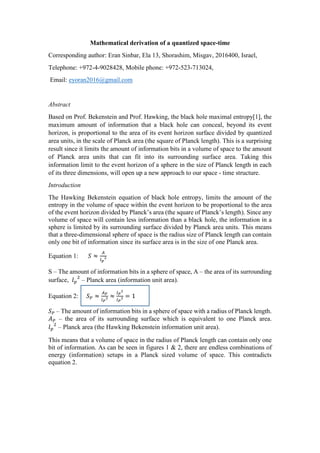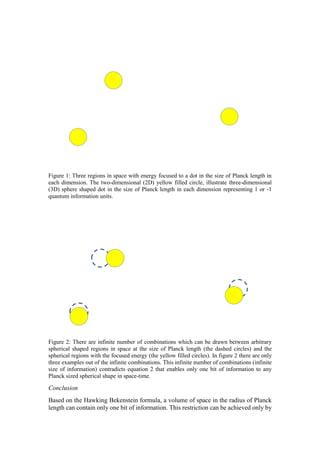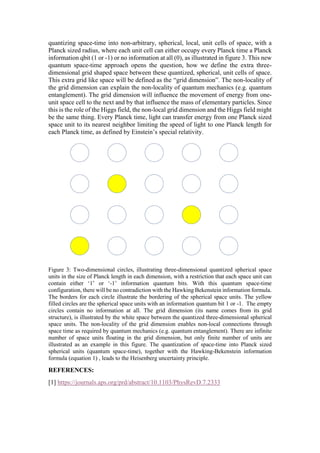Successfully reported this slideshow.

# Mathematical derivation of a quantized space-time.pdf×

# Mathematical derivation of a quantized space-time.pdf

Based on Prof. Bekenstein and Prof. Hawking, the black hole maximal entropy, the maximum amount of information that a black hole can conceal, beyond its event horizon, is proportional to the area of its event horizon surface divided by quantized area units, in the scale of Planck area (the square of Planck length). This is a surprising result since it limits the amount of information bits in a volume of space to the amount of Planck area units that can fit into its surrounding surface area. Taking this information limit to the event horizon of a sphere in the size of Planck length in each of its three dimensions, will open up a new approach to our space - time structure. Prof Bekenstein published 50 years ago his paper about " Black holes and entropy" .This paper proves mathematically , based on Prof. Bekenstein's formula that space-time must be quantized and this leads to the the discovery of an extra grid shaped dimension between the quantized units of space-time. This is a break through towards the ultimate connection between gravity and quantum mechanics in these days when physics is so blurry and confusing.

Based on Prof. Bekenstein and Prof. Hawking, the black hole maximal entropy, the maximum amount of information that a black hole can conceal, beyond its event horizon, is proportional to the area of its event horizon surface divided by quantized area units, in the scale of Planck area (the square of Planck length). This is a surprising result since it limits the amount of information bits in a volume of space to the amount of Planck area units that can fit into its surrounding surface area. Taking this information limit to the event horizon of a sphere in the size of Planck length in each of its three dimensions, will open up a new approach to our space - time structure. Prof Bekenstein published 50 years ago his paper about " Black holes and entropy" .This paper proves mathematically , based on Prof. Bekenstein's formula that space-time must be quantized and this leads to the the discovery of an extra grid shaped dimension between the quantized units of space-time. This is a break through towards the ultimate connection between gravity and quantum mechanics in these days when physics is so blurry and confusing.